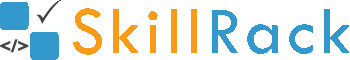Must Solve 100 Programs for Campus Placement Part - 1/104 months ago

1) Print SkillRack as the Output

The program must print SkillRack as the output.

Note: S and R are in upper case.

Click the link below to solve this program
http://www.skillrack.com/solve/7864

2) Print Skill Rack in first two lines

The program must print Skill in the first line and Rack in the second line.

Note: S and R are in upper case.

Output:
Skill
Rack

Click the link below to solve this program
http://www.skillrack.com/solve/7866

Accept two numbers and print their sum.

Example Input/Output 1:
Input:
10 20

Output:
30

Example Input/Output 2:
Input:
137 11

Output:
148

Click the link below to solve this program
http://www.skillrack.com/solve/7867

4) Multiply by 2

The program must accept a number and multiply it by 2.

Example Input/Output 1:
Input:
10

Output:
20

Example Input/Output 2:
Input:
50

Output:
100

Click the link below to solve this program
http://www.skillrack.com/solve/7868

5) Subtract Two Numbers

The program must accept two numbers and subtract them.

Example Input/Output 1:
Input:
100 40

Output:
60

Example Input/Output 2:
Input:
50 200

Output:
-150

Click the link below to solve this program
http://www.skillrack.com/solve/7869

6) Print Square

The program must accept a number and print it's square.

Example Input/Output 1:
Input:
5

Output:
25

Example Input/Output 2:
Input:
9

Output:
81

Click the link below to solve this program
http://www.skillrack.com/solve/7870

7) Print the Previous Number

The program must accept a number and print its previous number.

Example Input/Output 1:
Input:
68

Output:
67

Example Input/Output 2:
Input:
100

Output:
99

Click the link below to solve this program
http://www.skillrack.com/solve/7871

8) Print the Next Number

The program must accept a number and print its next number.

Example Input/Output 1:
Input:
5

Output:
6

Example Input/Output 2:
Input:
99

Output:
100

Click the link below to solve this program
http://www.skillrack.com/solve/7872

9) Print Cube

The program must accept a number and print it's cube.

Example Input/Output 1:
Input:
5

Output:
125

Example Input/Output 2:
Input:
3

Output:
27

Click the link below to solve this program
http://www.skillrack.com/solve/7873

Accept three numbers and print their sum.

Example Input/Output 1:
Input:
5 2 4

Output:
11

Example Input/Output 2:
Input:
25 15 10

Output:
50

Click the link below to solve this program
http://www.skillrack.com/solve/7874